# Polynomials

## Producer Field Guide

HGD_Product
Producer Field Guide
HGD_Portfolio_Suite
Producer

A polynomial is a mathematical expression consisting of variables and coefficients. A coefficient is a constant, which is multiplied by a variable in the expression.

Order

The variables in polynomial expressions can be raised to exponents. The highest exponent in a polynomial determines the order of the polynomial.

A polynomial with one variable, x, takes this form: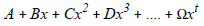Where: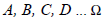= coefficients

t = order of the polynomial

If one or all of A, B, C, D ... are 0, then the nature, but not the complexity, of the transformation is changed. Mathematically,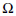cannot be 0.

A polynomial with two variables, x and y, takes this form: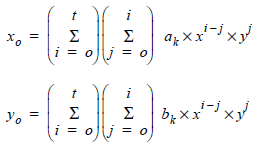Where:

t = order of the polynomial

ak and bk = coefficients

Subscript k is determined by: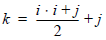A numerical example of 3rd-order transformation equations for x and y is: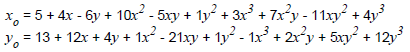Polynomial equations are used in image rectification to transform the coordinates of an input file to the coordinates of another system. The order of the polynomial used in this process is the order of transformation.

Transformation Matrix

In the case of first order image rectification, the variables in the polynomials (x and y) are the source coordinates of a GCP. The coefficients are computed from the GCPs and stored as a transformation matrix.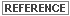A detailed discussion of GCPs, orders of transformation, and transformation matrices is included in Rectification.